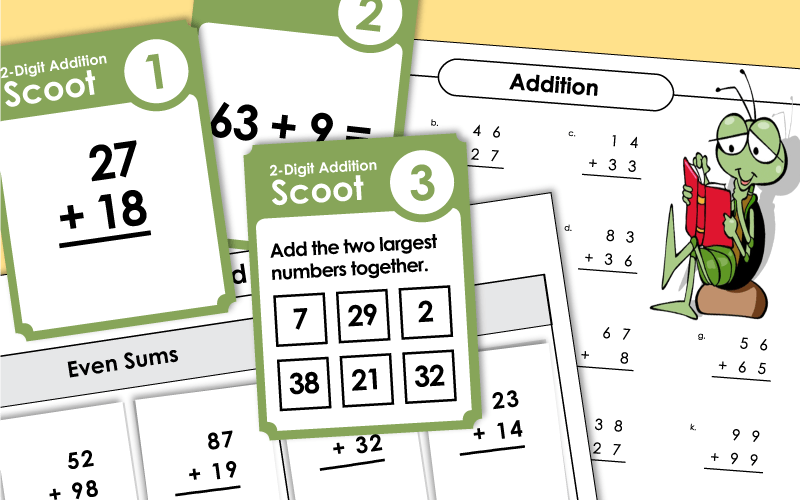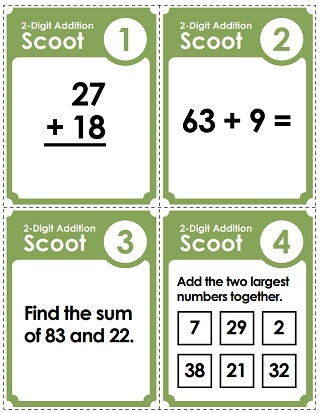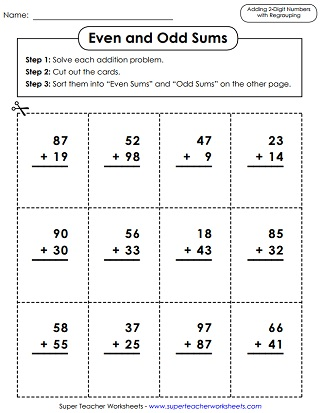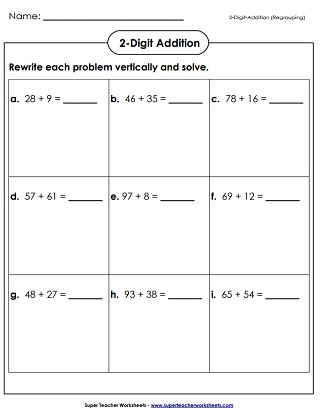Your whole class can participate in this fast-paced game. Students move around the room, from desk-to-desk solving two-digit addition problems. All problems require students to know how to regroup.
This file has 30 task cards. Each one has a 2-digit addition problem on it. These can be used for classroom games or learning centers.

Add the numbers together starting with the ones place. Regroup if necessary and show the regrouping with the box provided. After, circle whether you regrouped or not.
Solve these addition problems with 2-digit addends. This worksheet DOES require regrouping.
Find the sums of these 2-digit by 2-digit addition problems. Problems do require regrouping (carrying). This worksheet features a pictures of a dog and two canine word problems.
Solve these double-digit addition word problems. These problems DO require regrouping.
Students are shown a set of numbers written inside of various shapes. They add similar shapes together. For example: Add the numbers in the trapezoids.
Print out these addition pennants, have each student make a pennant by solving the addition problem, coloring the border, and cutting it out, and glue the pennants along a rope.
This worksheet has addition problems that were not solved correctly. Students must find and explain the errors.
Kids will be able to follow the place-value columns easily when they add on this graph paper; 2-digits plus 2-digits.
This worksheet helps students use place value blocks (base 10 locks) to learn addition.
Each of the nine addition problems on this page is written horizontally. Students rewrite each one vertically to solve.
First, solve the addition problems. Then cut out the problem cards and sort them into two categories on the t-chart: Odd Sums and Even Sums.
Solve each 2-digit addition problem. Explain why you did or did not carry the one in each problem.
Add the double-digit numbers. Cut out the puzzle pieces. Place each puzzle piece on the correct square with the corresponding sum. The finished puzzle picture shows a rocket traveling through outer space.
Add the number on the quarterback's jersey to the number on the receiver's jersey to complete each pass. This 2-page worksheet includes 6 problems.
Choose numbers from the circles and add them together. Add six pairs.
This worksheet includes a few different styles of questions. Some problems require regrouping.

Use each set of digits to make addition problems. This file has includes a set of task cards.
On this worksheet, students must balance the scale by writing in missing addends or sums. Note: This may be a tricky activity for younger students.
Use subtraction to find the missing addend in each equation.
Find the missing addends to calculate the number of years your team needs to score a touchdown.

Practice finding sums of three, two-digit addends. This PDF has 12 problems, including a word problem.
These addition problems have already been solved. Look carefully for mistakes. Explain the errors and solve correctly.

With this online generator, you'll be able to make customized addition worksheets with 2, 3, 4, 5, 6, or 7 digits. You'll also be able to create your own heading and title, as well as a customized footer.

The link above will take you to a page with basic addition facts (0-10). Includes flashcards, games, timed quizzes, and learning centers.

This page has math addition worksheets that do NOT require borrowing or regrouping. Most worksheets have double digit problems. Some have triple digits.

Here's our collection of triple-digit addition worksheets. (Approximate grade levels: 2nd and 3rd)

## Sample Worksheet ImagesMy Account
Site Information